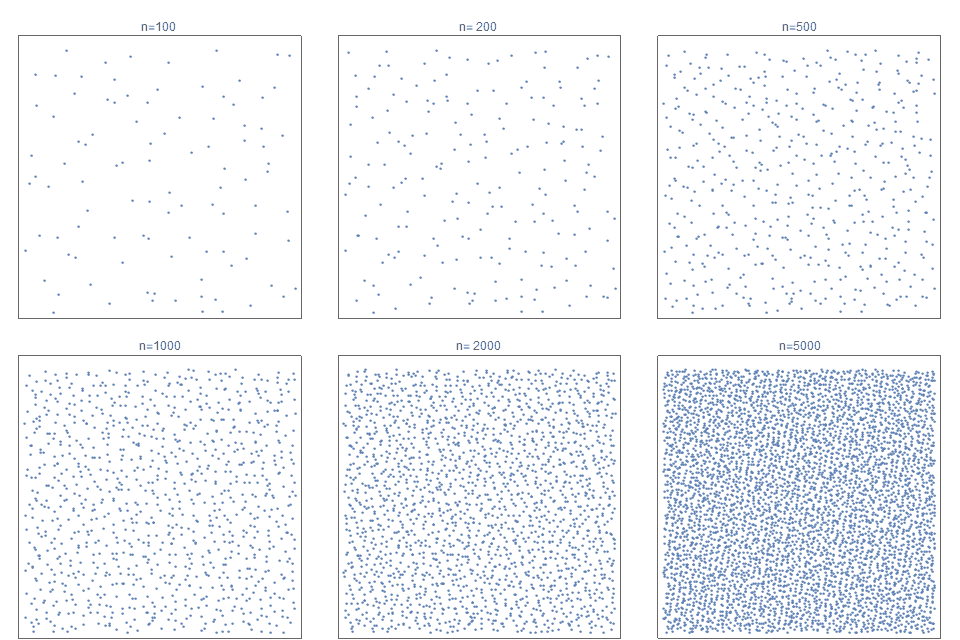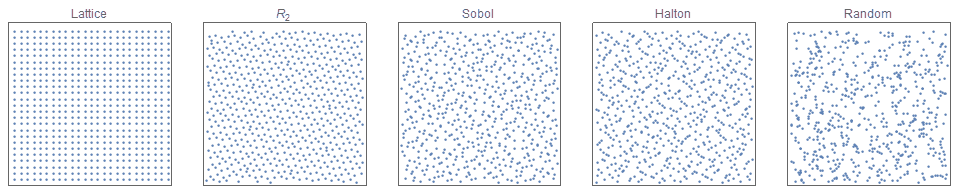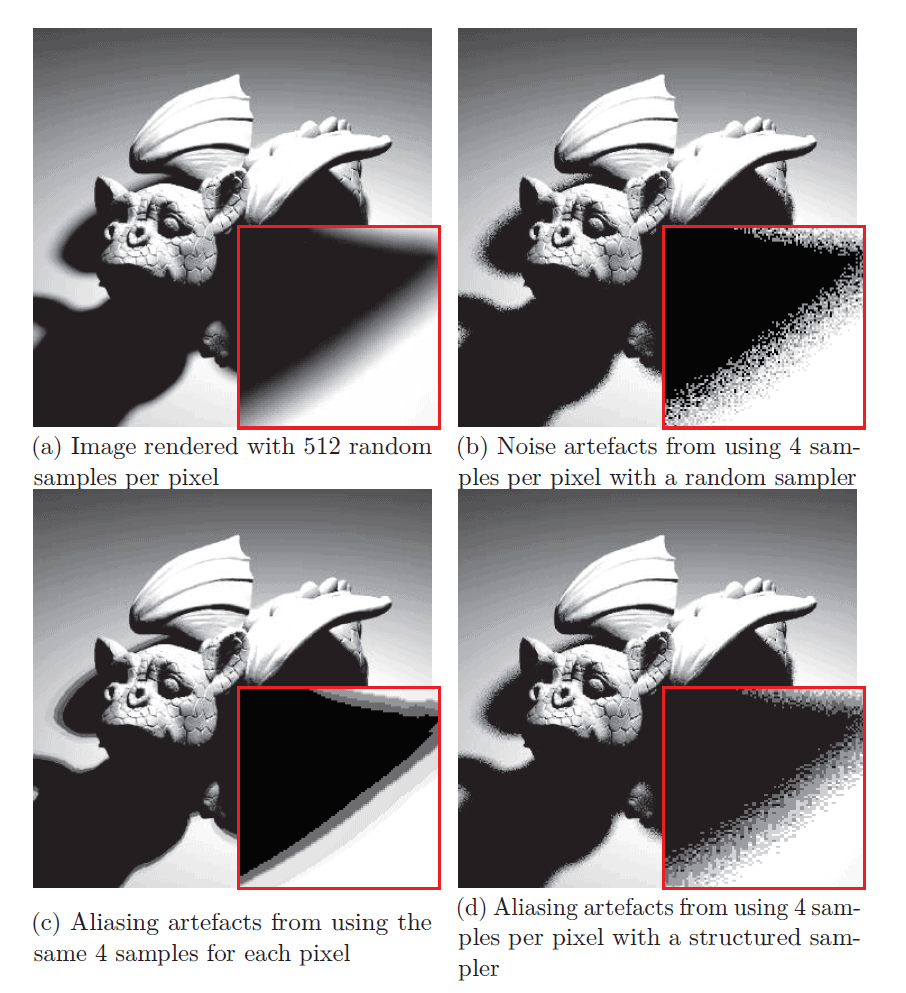# A simple method to construct isotropic quasirandom blue noise point sequences

I describe a simple method for constructing a sequence of points, that is based on a low discrepancy quasirandom sequence but exhibits enhanced isotropic blue noise properties. This results in fast convergence rates with minimal aliasing artifacts.Figure 1. The first 100, 200, 500, 1000, 2000 and 5000 sample points of the proposed point sequence (eqn 11) that is progressive, non-stochastic, exhibits near isotropic blue noise characteristics with fast QMC convergence rates with reduced artifacts. It is based on a new simple low discrepancy quasirandom sequence, $R_2$.

First published: 13th November, 2018 Last updated:    2nd March, 2020

# Introduction

Quasirandom low discrepancy sequences are useful to create distributions that appear less regular then lattices, but more regular than simple random distributions (see figure 2). They play a large role in many numerical computing applications, including physics, finance and in more recent decades computer graphics.Figure 2. Comparison of a regular lattice (left) with 3 different quasirandom functions (middle), and a simple random distribution (right). Notice that the quasirandom distributions appear less regular than a lattice but do not have as many ‘clumps’ or ‘gaps’ as the random distribution.

The  classic paper on how using quasirandom sampling over uniform sampling can improve 2D images is “Stochastic Sampling in Computer Graphics” [Cook, 1986]. In more recent decades, quasirandom sampling has become a major tool in 3D rendering. Rendering (and samplers) can utilize either the CPU or GPU for calculations. For an excellent primer on their use in 3D rendering, see “Anti-aliased Low Discrepancy Samplers for Monte Carlo Estimators in Physically Based Rendering” [Perrier, 2108]. In this paper, she describes the task of 3D rendering (see figure 3) as follows:

“When displaying a 3-D object on a computer screen, this 3-D scene is turned into a 2-D image, which is a set of organized colored pixels. We call Rendering the process that aims at finding the correct color to give those pixels. This is done by simulating and integrating all the light,….we numerically approximate the equation by taking random samples in the integration domain and approximating the integrand value using Monte Carlo methods….A stochastic sampler should minimize the variance in integration to converge to a correct approximation using as few samples as possible. Many different samplers exist that we roughly regroup into two families: –  Blue Noise samplers, that have a low integration variance while generating unstructured point sets. The issue with those samplers is that they are often slow to generate a point set – Low Discrepancy samplers, that minimize the variance in integration and are able to generate and enrich a point set very quickly. However, they present a lot of structural artifacts when used in Rendering.”

In recent years, efforts have been made to combine to the anti-aliasing advantages of jittered sampling, with the fast convergence rates (low variance) associated with low discrepancy quasirandom sequences [Christensen, 2018]. With this same goal, I present a new and different method to construct an infinite point sequence, that is extremely  simple,  fast to calculate, progressive fully deterministic, exhibits fast convergence rates (low variance), with desirable isotropic, blue noise spectra and therefore results in minimal artifacts

So here goes…Figure 3. Rendering in 3D (left). Undesirable aliasing artifacts often arise in sampling processes (right). Source:[Perrier, 2018]

# Outline

• simple jittered (finite) point sets
• jittered quasirandom point sets
• jittering of (infinite) quasirandom sequences
• QMC convergence comparisons
• summary
• example code

# Simple Jittered Sampling

The most basic jittered (perturbed) point sets are the jittered grids. In this case, the sample space is subdivided into a grid of $m \times m$ square regions. Instead of merely selecting a point at the center of each cell, any (pseudo-) random point within each cell is selected.The advantages of jittered grid sampling is that it is trivial to understand,  simple to implement, and extremely fast to compute. It has the obvious limitation that $N$ must be a perfect square.

Although rectangular stratified sampling can ease this limitation by simply requiring $N$ to be the product of two appropriate integers, I describe a different approach that is is informative, very elegant but less well known. Without loss of generality, assume that our sample space is the unit square $[0,1)^2$.

We define the Fibonacci lattice as of $N$ points where:

$$\pmb{p}_i(x,y) = \left( \frac{i-0.5}{N}, \left\{ \frac{i}{\phi} \right\}\; \right); \quad \textrm{for } \; i=1,2,3,…, N. \tag{1}$$